Question

# A long wire carrying 6.50 A of current makes two bends, as shown in the figure.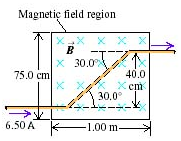A long wire carrying 6.50 A of current makes two bends, as shown in the figure. The bent part of the wire passes through a uniform 0.280 T magnetic field directed as shown in the figure and confined to a limited region of space.

A) Find the magnitude of the force that the magnetic field exerts on the wire.

B) Find the direction of the force that the magnetic field exerts on the wire.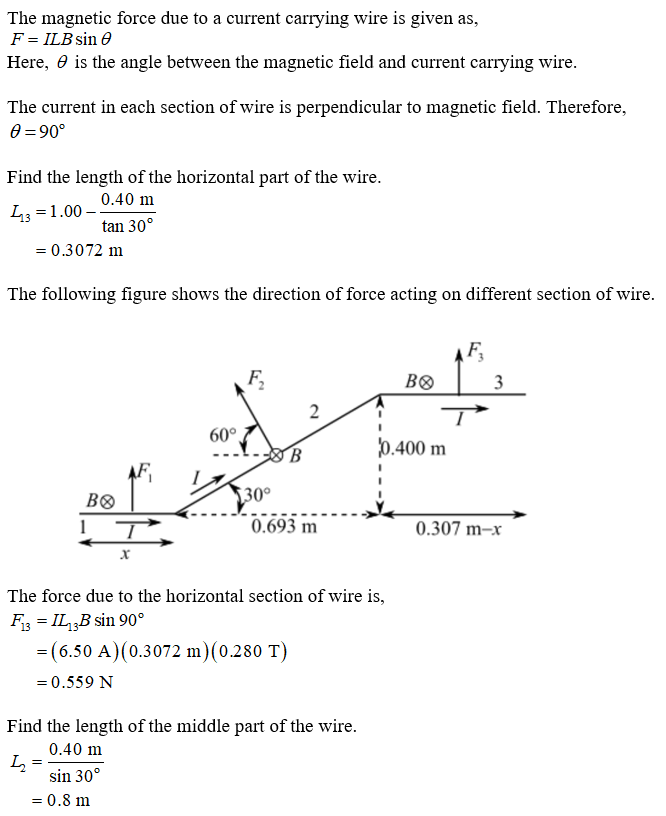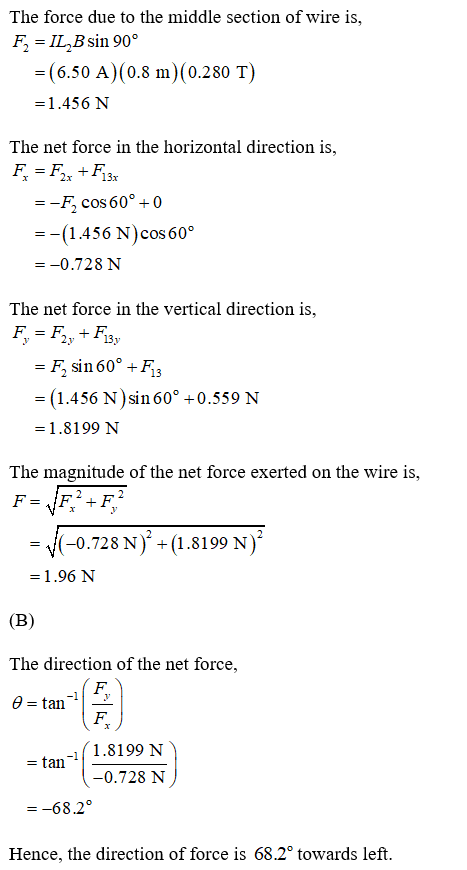#### Earn Coins

Coins can be redeemed for fabulous gifts.

Similar Homework Help Questions
• ### ​A long wire carrying 6.50 A of currents makes two bends, as shown in the figure on the right.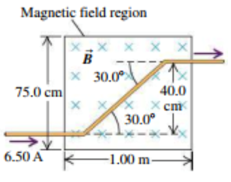A long wire carrying 6.50 A of currents makes two bends, as shown in the figure on the right. The bent part of the wire passes through a uniform 0.280-T magnetic field directed as shown and confined to a limited region of space. a. What are the magnitude and direction of the force exerted on the wire by the magnetic field?b. Now assume that the magnetic field extends 1.00 m to the left and to the right of the region shown...

• ### A long wire carrying 4.50 A of current makes two 90 bends, as shown in the...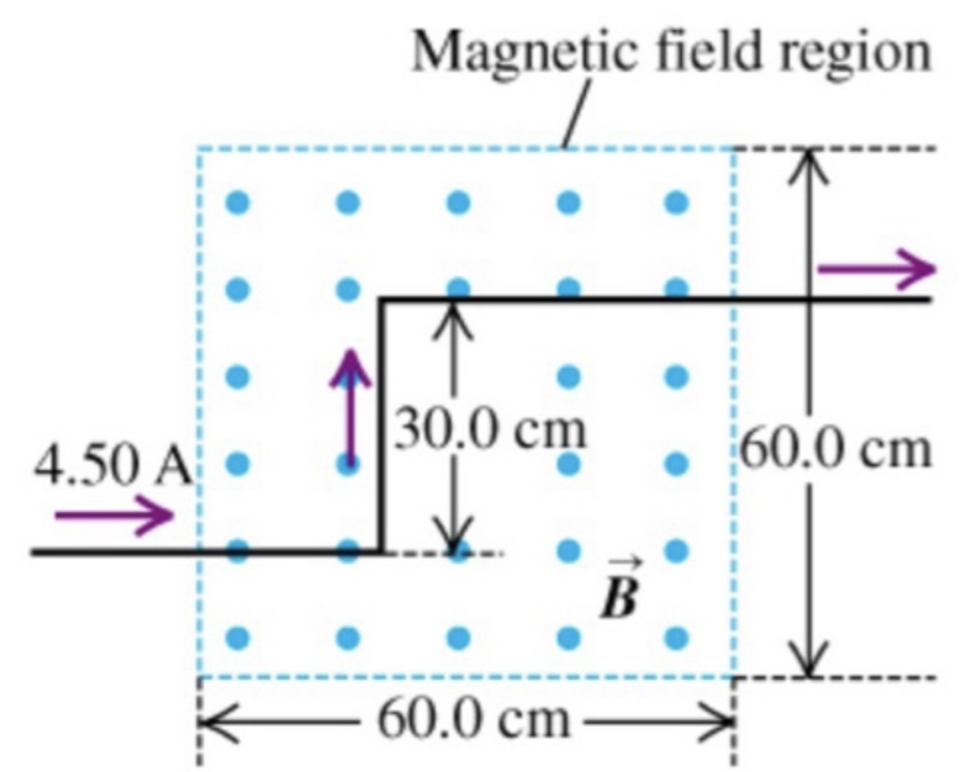A long wire carrying 4.50 A of current makes two 90° bends, as shown in the figureThe bent part of the wire passes through a uniform 0.238 T magnetic field directed as shown in the figure and confined to a limited region of space.Part AFind the magnitude of the force that the magnetic field experts on the the wire.Part BFind the direction of the force that the magnetic field experts on the the wire.

• ### A long wire carrying 4.50 A of current makes two 90 degree bends, as shown in....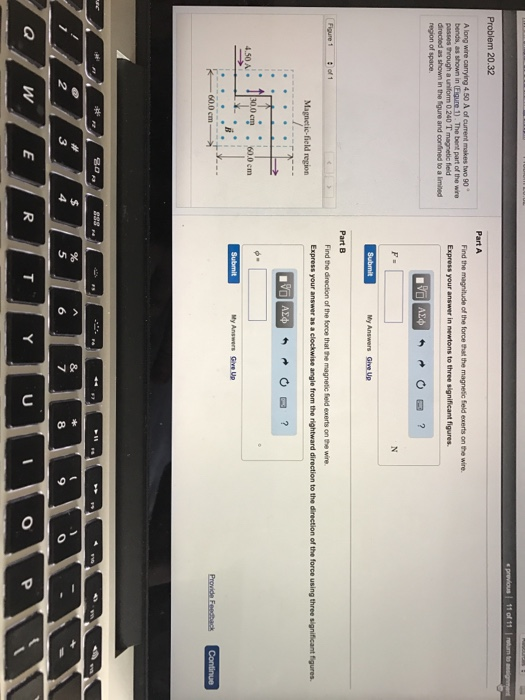A long wire carrying 4.50 A of current makes two 90 degree bends, as shown in. The best part of the wire passes through a uniform 0.240 t magnetic field directed as shown in the figure and confined to a limited of space. Part A Find the magnitude of the force that the magnetic field exerts on the wire Part B Find the direction of the force that the magnetic field exerts on the wire.

• ### Problem 4 Magnetic field region Fig. 2 A long wire carrying 4.50 A of current makes...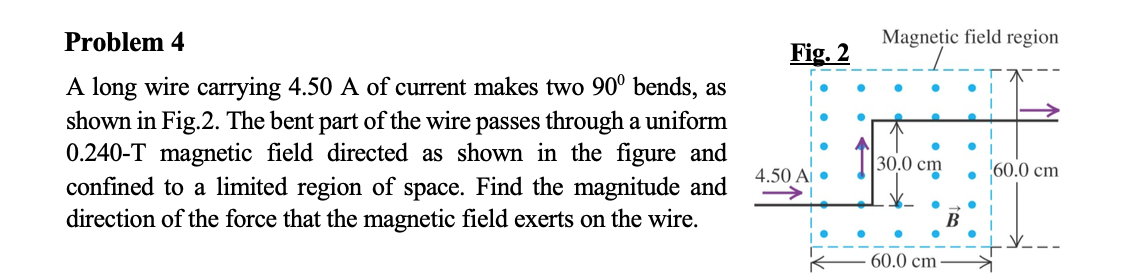Problem 4 Magnetic field region Fig. 2 A long wire carrying 4.50 A of current makes two 90° bends, as shown in Fig.2. The bent part of the wire passes through a uniform 0.240-T magnetic field directed as shown in the figure and confined to a limited region of space. Find the magnitude and direction of the force that the magnetic field exerts on the wire. . ||30.0 cm 60.0 cm 4.5 Y_ - 60.0 cm

• ### A long wire carrying a 6.00A current reverses direction by means of two right-angle bends, as...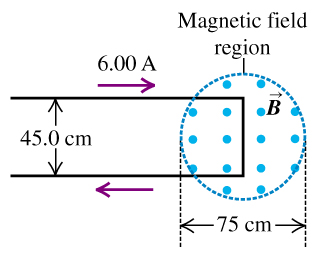A long wire carrying a 6.00A current reverses direction by means of two right-angle bends, as shown in the figure (Figure 1) . The part of the wire where the bend occurs is in a magnetic field of 0.666T confined to the circular region of diameter 75cm, as shown. A) Find the direction of the net force that the magnetic field exerts on this wire. B) Find the magnitude of the net force that the magnetic field exerts on this...

• ### A wire carrying a 31.0 A current bends through a right angle.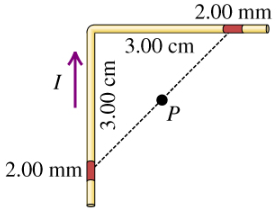Exercise 28.13 - Enhanced - with Solution A wire carrying a 31.0 A current bends through a right angle. Consider two 2.00 mm segments of wire, each 3.00 cm from the bend (Figure 1). Part A Find the magnitude of the magnetic field these two segments produce at point P, which is midway between them. Express your answer with the appropriate units. Part B Find the direction of the magnetic field at point P. out of the page into the page

• ### The bent wire shown in the figure lies in a uniform magnetic field. Each straight section is 2.0 m long and makes an angle of θ = 60° with the x axis, and the wire carries a current of 2.0 A.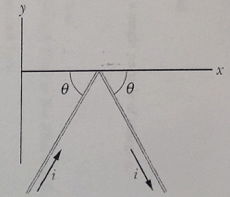The bent wire shown in the figure lies in a uniform magnetic field. Each straight section is 2.0 m long and makes an angle of θ = 60° with the x axis, and the wire carries a current of 2.0 A. What is the magnitude of the magnetic force on the wire in unit-vector notation if the magnetic field is given by (a) 4.0 k T and (b) 4.0 iT?

• ### (​Magnetic Force on a Wire​) A horizontal, current-carrying wire of mass m = 40.0 g is...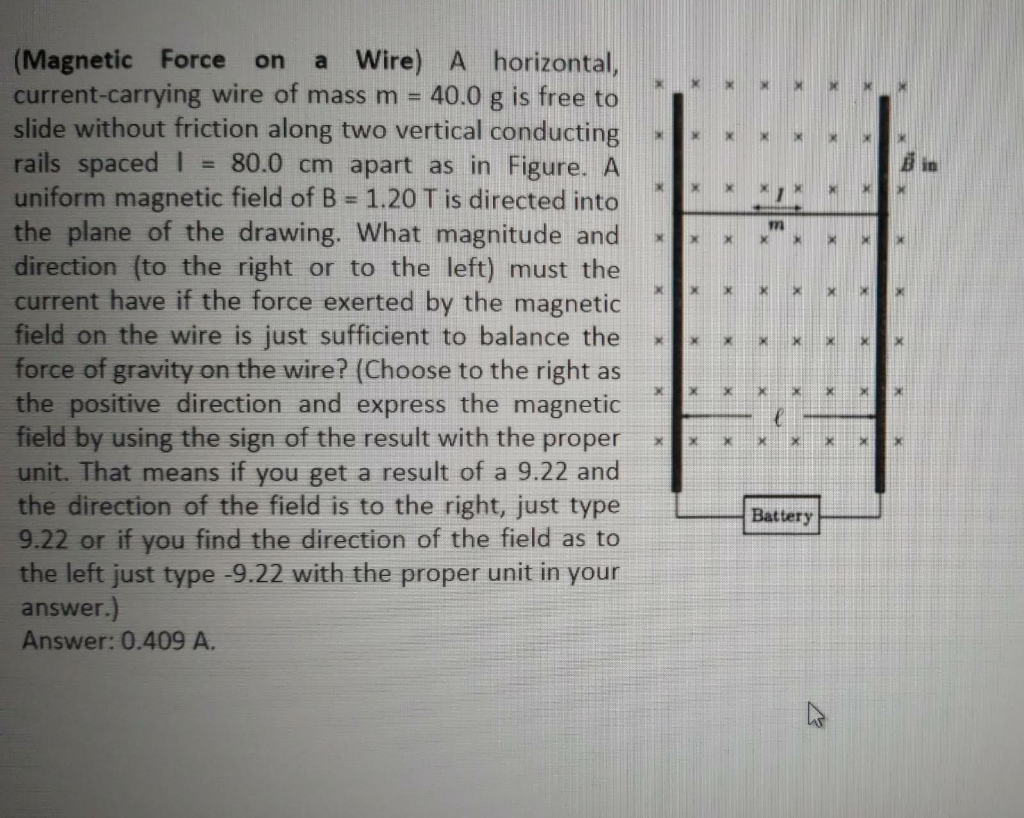(​Magnetic Force on a Wire​) A horizontal, current-carrying wire of mass m = 40.0 g is free to slide without friction along two vertical conducting rails spaced l = 80.0 cm apart as in Figure. A uniform magnetic field of B = 1.20 T is directed into the plane of the drawing. What magnitude and direction (to the right or to the left) must the current have if the force exerted by the magnetic field on the wire is just...

• ### QUESTION # 01 Above figure shows a wire carrying a current in the presence of a...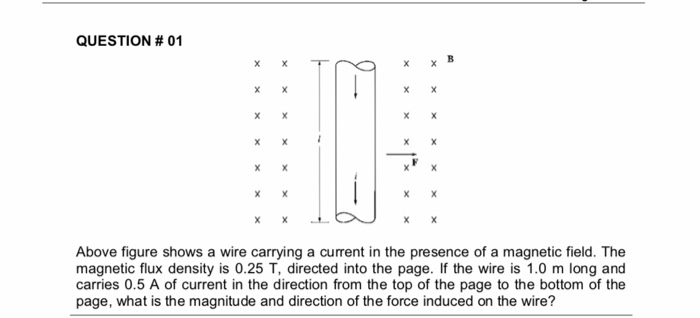QUESTION # 01 Above figure shows a wire carrying a current in the presence of a magnetic field. The magnetic flux density is 0.25 T, directed into the page. If the wire is 1.0 m long and carries 0.5 A of current in the direction from the top of the page to the bottom of the page, what is the magnitude and direction of the force induced on the wire?